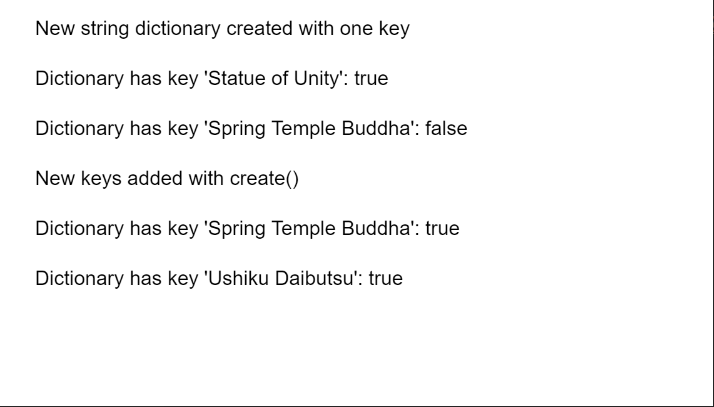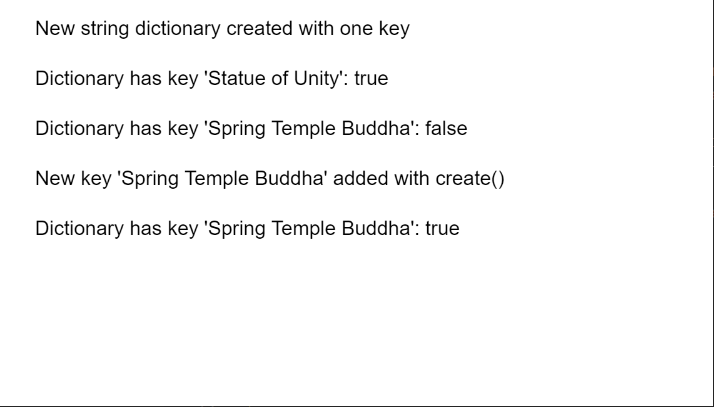# p5.js TypedDict create() Method

• Last Updated : 25 Nov, 2020

The create() method of p5.TypedDict in p5.js is used to add the given key-value pair or collection of pairs to the dictionary. A key-value pair is a set of two values that are mapped to each other. These values can be accessed by querying this dictionary using the key portion of the pair. A dictionary can store multiple key-value pairs that can be accessed using the methods of the dictionary.

Syntax:

Hey geek! The constant emerging technologies in the world of web development always keeps the excitement for this subject through the roof. But before you tackle the big projects, we suggest you start by learning the basics. Kickstart your web development journey by learning JS concepts with our JavaScript Course. Now at it's lowest price ever!

```create( key, value )
```

or

```create( obj )
```

Parameters:

• key: It specifies the string that is used as the key to be added to the dictionary.
• value:It specifies the string that is used as the value to be added to the dictionary.
• obj: It specifies the object that contains the key-value pairs to be added to the dictionary.

The example below illustrates the create() method in p5.js:

Example 1:

## Javascript

 `function` `setup() {``  ``createCanvas(550, 300);``  ``textSize(16);`` ` `  ``let stringDict =``      ``createStringDict(``"Statue of Unity"``, ``"182 m"``);``  ``text(``"New string dictionary created "` `+``       ``"with one key"``, 20, 20);`` ` `  ``let existOne = ``      ``stringDict.hasKey(``"Statue of Unity"``);``  ``text(``"Dictionary has key "` `+``       ``"'Statue of Unity': "` `+``       ``existOne, 20, 60);`` ` `  ``let existTwo = ``      ``stringDict.hasKey(``"Spring Temple Buddha"``);``  ``text(``"Dictionary has key "` `+``       ``"'Spring Temple Buddha': "` `+``       ``existTwo, 20, 100);`` ` `  ``let tmpObj = {``    ``"Spring Temple Buddha"``: ``"128 m"``,``    ``"Ushiku Daibutsu"``: ``"100 m"``,``    ``"Great Buddha of Thailand"``: ``"92m"``  ``};`` ` `  ``// Add the given key to the dictionary``  ``// specifying the key and value as an object``  ``stringDict.create(tmpObj);``  ``text(``"New keys added with create()"``,``       ``20, 140);`` ` `  ``existTwo = ``    ``stringDict.hasKey(``"Spring Temple Buddha"``);``  ``text(``"Dictionary has key "` `+``       ``"'Spring Temple Buddha': "` `+``       ``existTwo, 20, 180);`` ` `  ``let existThree =``      ``stringDict.hasKey(``"Ushiku Daibutsu"``);``  ``text(``"Dictionary has key "` `+``       ``"'Ushiku Daibutsu': "` `+ ``       ``existThree, 20, 220);``}`

Output:Example 2:

## Javascript

 `function` `setup() {``  ``createCanvas(550, 300);``  ``textSize(16);`` ` `  ``let stringDict =``      ``createStringDict(``'Statue of Unity'``,``                       ``'182 m'``);``  ``text(``"New string dictionary "` `+``       ``"created with one key"``, 20, 20);`` ` `  ``let existOne =``      ``stringDict.hasKey(``'Statue of Unity'``);``  ``text(``"Dictionary has key 'Statue of Unity': "``        ``+ existOne, 20, 60);`` ` `  ``let existTwo =``      ``stringDict.hasKey(``'Spring Temple Buddha'``);``  ``text(``"Dictionary has key "` `+``       ``"'Spring Temple Buddha': "` `+``       ``existTwo, 20, 100);`` ` `  ``// Add the given key to the dictionary``  ``// specifying the key and value``  ``stringDict.create(``'Spring Temple Buddha'``,``                    ``'128 m'``);``  ``text(``"New key 'Spring Temple Buddha'"` `+``       ``" added with create()"``, 20, 140)`` ` `  ``existTwo = ``    ``stringDict.hasKey(``'Spring Temple Buddha'``);``  ``text(``"Dictionary has key "` `+``       ``"'Spring Temple Buddha': "` `+``       ``existTwo, 20, 180);``}`

Output:Online editor: https://editor.p5js.org/
Environment Setup: https://www.geeksforgeeks.org/p5-js-soundfile-object-installation-and-methods/
Reference: https://p5js.org/reference/#/p5.TypedDict/create

My Personal Notes arrow_drop_up